Summation Notation

Summation Notation is a mathematical notation for finding the sum of the terms of any sequence. The mathematical symbol for summation is the Greek letter sigma,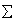. In the previous book we used the following example to denote the sum of the first five terms of a sequence: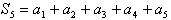. The notation that describes the summation of the first term through the fifth term of a sequence is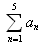. Therefore,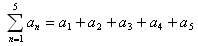.

The notation for the sum of n terms is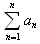. In summation notation, the letter n is called the index of summation . The value below the sigma represents the initial term. The value above the sigma represents the final term. an represents the explicit formula for the sequence.